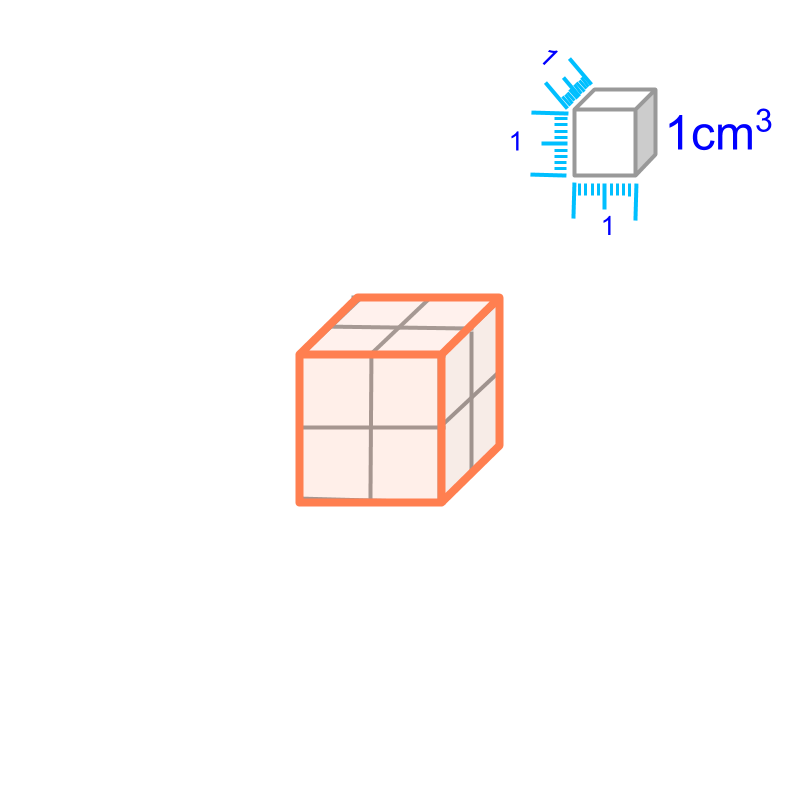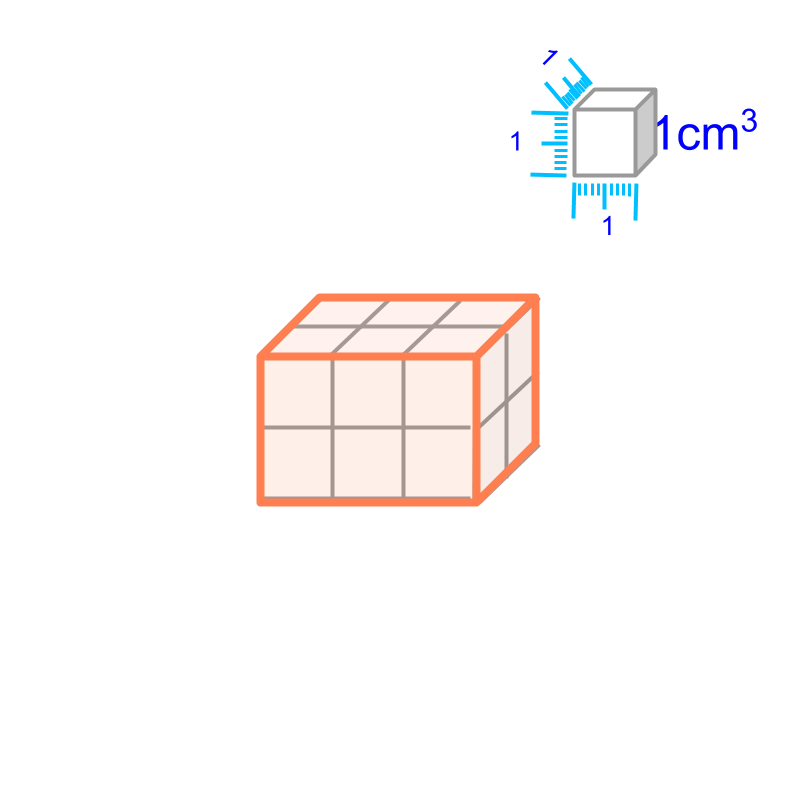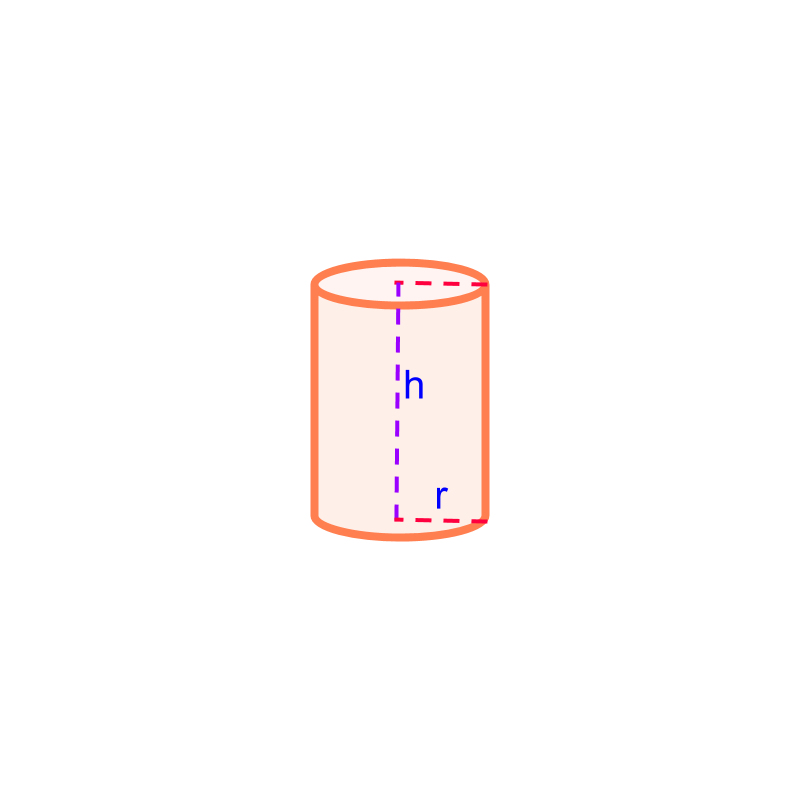maths > mensuration-basics

Volume : Cube, Cuboid, Cylinder

what you'll learn...

Overview

Volume of Some Solids :Volume of cube $={a}^{3}$$= {a}^{3}$Volume of cuboid $=lbh$$= l b h$Volume of cylinder $=\pi {r}^{2}h$$= \pi {r}^{2} h$

volume

Volume is the "space-span within a closed 3D-solid".The volume of a cube of side $a$$a$ is "${a}^{3}$${a}^{3}$".The volume of a cuboid of length $l$$l$, breadth $b$$b$, and height $h$$h$ is "$lbh$$l b h$".The volume of a cylinder of height $h$$h$ and radius $r$$r$ is the area of cross section multiplied the height, given by $\pi ×{r}^{2}×h$$\pi \times {r}^{2} \times h$

What is the volume of a cuboid of length $2$$2$ cm, breadth $3$$3$ cm and height $4$$4$ cm?
The answer is "$24c{m}^{3}$$24 c {m}^{3}$".

summary

Volume of Some Solids :Volume of cube $={a}^{3}$$= {a}^{3}$Volume of cuboid $=lbh$$= l b h$Volume of cylinder $=\pi {r}^{2}h$$= \pi {r}^{2} h$

Outline

The outline of material to learn "Mensuration basics : Length, Area, & Volume" is as follows.

•  Measuring Basics

→   Introduction to Standards

→   Measuring Length

→   Accurate & Approximate Meaures

→   Measuring Area

→   Measuring Volume

→   Conversion between Units of Measure

•  2D shapes

→   Perimeter of Polygons

→   Area of Square & rectangle

→   Area of Triangle

→   Area of Polygons

→   Perimeter and area of a Circle

→   Perimeter & Area of Quadrilaterals

•  3D shapes

→   Surface Area of Cube, Cuboid, Cylinder

→   Volume of Cube, Cuboid, Cylinder